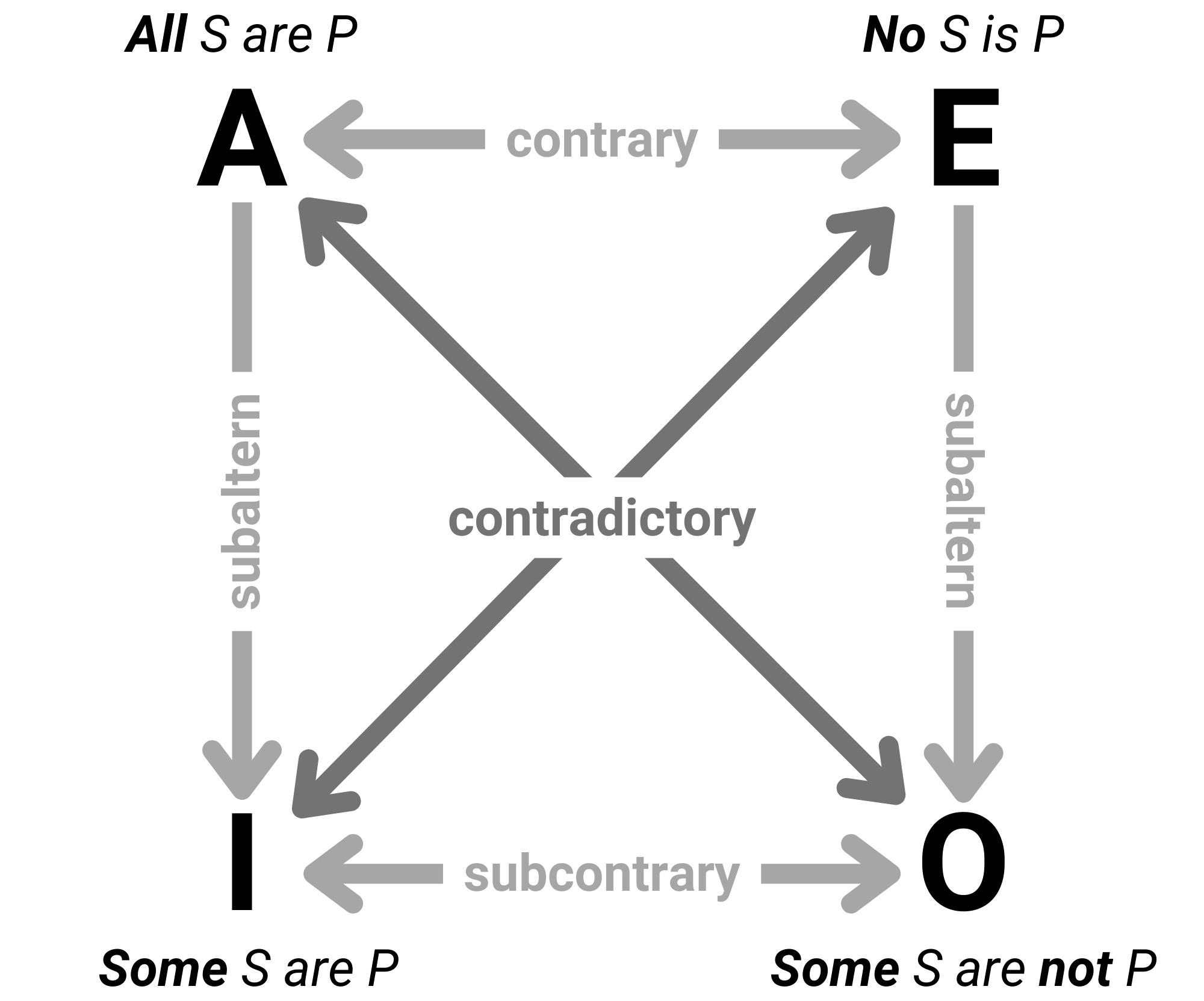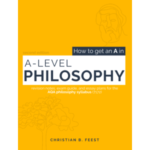# The Square of Opposition Explained

Dating back at least as far as Aristotle in the 4th Century BC, the Square of Opposition is a visual framework for representing the logical connections and relationships between four basic categorical propositions:

• A: All S are P
• E: No S is P
• I: Some S are P
• O: Some S are not P

These propositions express information about the classes or categories of objects or individuals. The square of opposition below represents how these propositions relate to each other:A proposition (All S are P) and O proposition (Some S are not P) are contradictories, as are E proposition and I proposition.

When propositions are contradictory, the truth of one proposition implies the falsity of the other and vice versa. In other words, if one is true the other must be false, and if one is false the other must be true.

For example:

• A: All cats are mammals
• O: Some cats are not mammals

If all cats are mammals (A proposition) is true, then it must be false that some cats are not mammals (O proposition). If one proposition is true, the other must be false.

And vice versa: If all cats are mammals (A proposition) were false, then it would be true that some cats are not mammals (O proposition).

Another example:

• E: No mammals can fly
• I: Some mammals can fly

Again, these propositions directly contradict each other: If no mammals can fly (E proposition), then it must be false that some mammals can fly (I proposition) and vice versa.

## 2. Contrary Pairs:

A proposition (All S are P) and E proposition (No S are P) are contraries.

When propositions are contrary, they cannot both be true simultaneously. In other words, if one is true, the other must be false.

However, although it’s impossible for contraries to both be true, it is possible for both contraries to be false.

For example:

• A: All birds can fly
• E: No birds can fly

In this example, both statements are false. A claims that all birds can fly, but we know there are flightless birds like penguins and ostriches, which makes this statement false. On the other hand, E asserts that no birds can fly, which is also false.

However, if A proposition were true (all birds could fly), then it would be impossible for E proposition to also be true (no birds can fly).

## 3. Subcontrary Pairs:

I proposition (Some S are P) and O proposition (Some S are not P) are subcontraries.

When propositions are subcontrary, they cannot both be false simultaneously. In other words, if one is false, the other must be true.

However, although it’s impossible for contraries to both be true, it is possible for both contraries to be true.

For example:

• I: Some cats are friendly
• E: Some cats are not friendly

In this example, both statements are true. I claims that some cats are friendly, and some cats are indeed friendly. E claims that some cats are not friendly, and this is also true.

## 4. Subalternation Pairs:

A proposition (All S are P) implies the corresponding I proposition (Some S are P). Similarly, E proposition (No S are P) implies the corresponding O proposition (Some S are not P). These pairs are known as subalterns.

When propositions are subalterns, the truth of the universal proposition implies the truth of the specific proposition.

However, notice that the arrow on the diagram only goes one way: The truth of the specific proposition (I or O) does not imply the corresponding universal proposition (A or E).

For example:

• A: All mammals have hair
• I: Some mammals have hair

In this example, if we accept the A proposition that all mammals have hair, the I proposition that some mammals have hair logically follows. So, the truth of the universal A proposition implies the corresponding particular I proposition.

However, all mammals have hair (A proposition) does not logically follow from some mammals have hair (I proposition).

Another example:

• E: No birds can swim
• O: Some birds can not swim

Again, the truth of the universal E proposition implies the truth of the specific O proposition: If it’s true that no birds can swim, then it’s true that some birds can’t swim.

And again, it doesn’t work the other way though: Just because some birds can’t swim (O proposition), it doesn’t necessarily mean no birds can swim (E proposition).The philosophy textbook written with the student in mind!